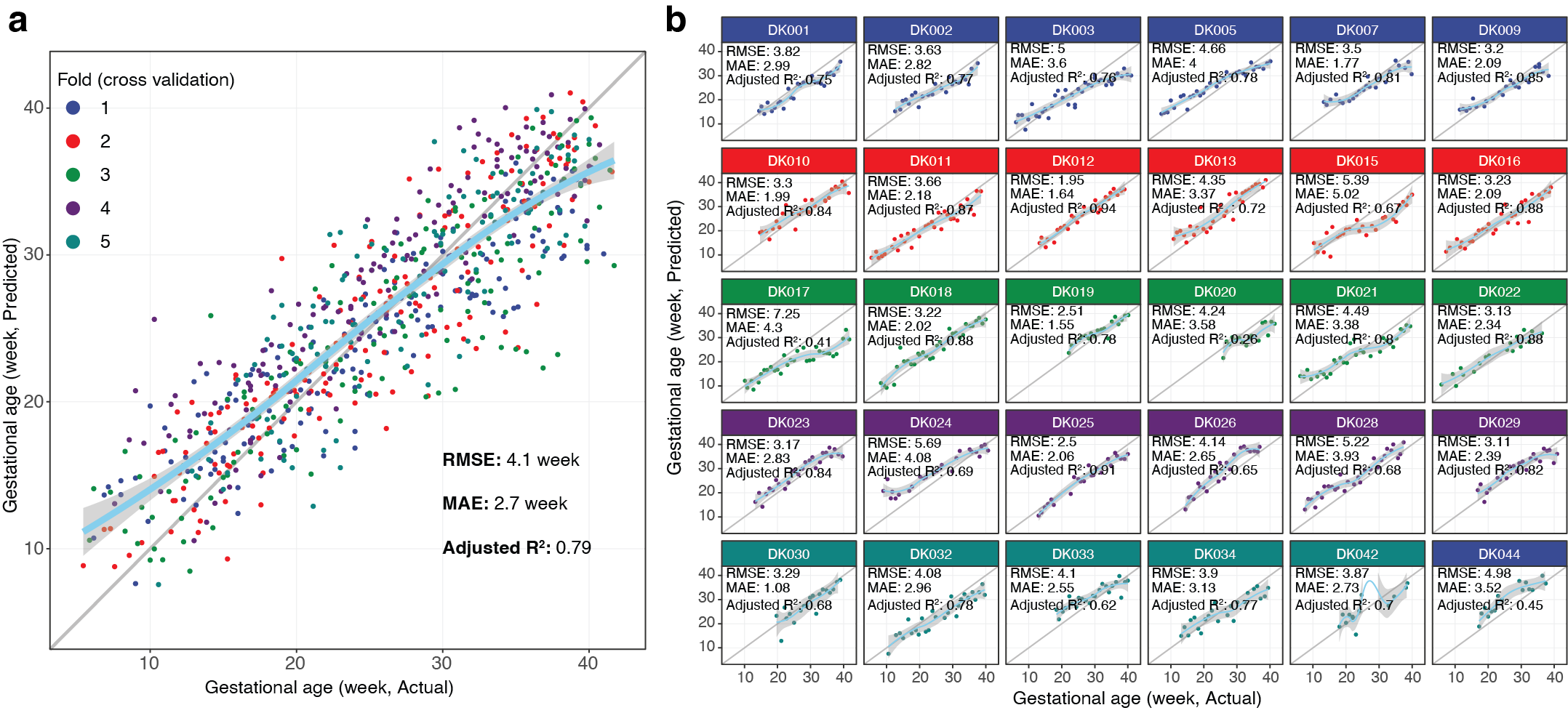# DeepPseduoMSI project

The deepPseudoMSI project is the first method that convert LC-MS raw data to “images” and then process them using deep learning method for diagnosis.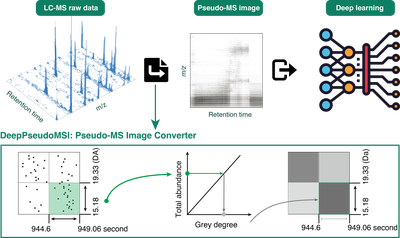# DeepPseudoMSI

Deep learning-based pseudo-mass spectrometry imaging analysis for precision medicine

The `deepPseudoMSI` project is the first method that convert LC-MS raw data to “images” and then process them using deep learning method for diagnosis. It contains two parts.

1. Pseudo-MS image converter

One LC-MS raw data usually contains millions of data points, so we need to divide it into different pixels (or grids) based on the revolution in the x-axis (RT) and y-axis (m/z) to reduce the size. All the data points in the same pixel are combined to represent the intensity of this pixel. The intensity of each pixel is linearly transformed to the color of the pixel (grey degree). Finally, one LC-MS raw data with millions of data points is converted into an image with thousands of pixels based on the resolution (for example, 224 × 224), we call it the pseudo-MS image, which contains all the information from the LC-MS raw data.

Code is here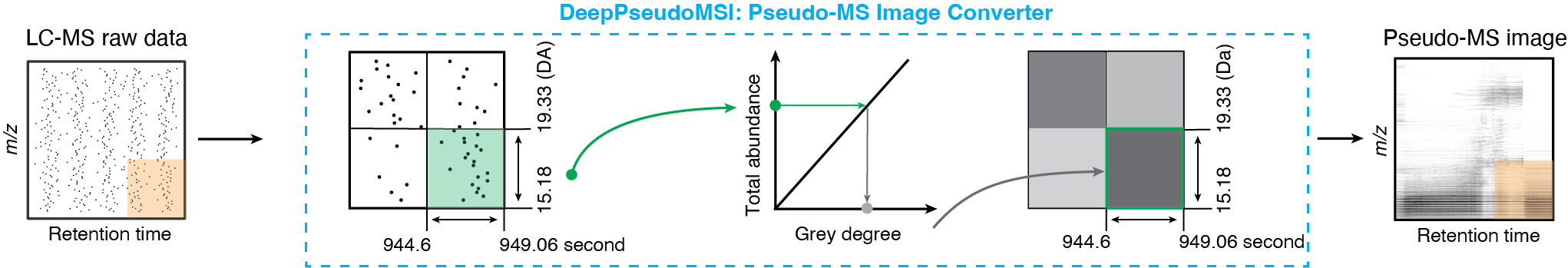2. Image predictor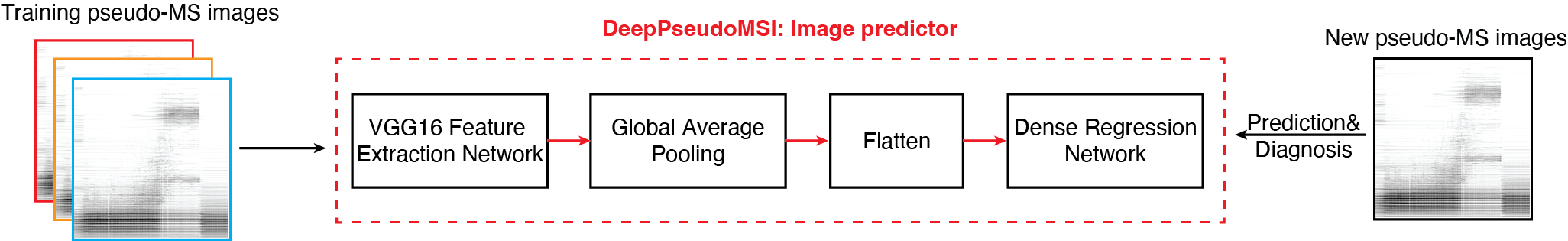# Case study

## Introduction

The case study dataset (RPLC, positive mode) from our previous published study to predict the gestational age (GA, week) of pregnant women (Liang et al, Cell, 2020). This study aims to predict the GA of pregnant women, so we could provide a non-invasive method for pregnancy dating.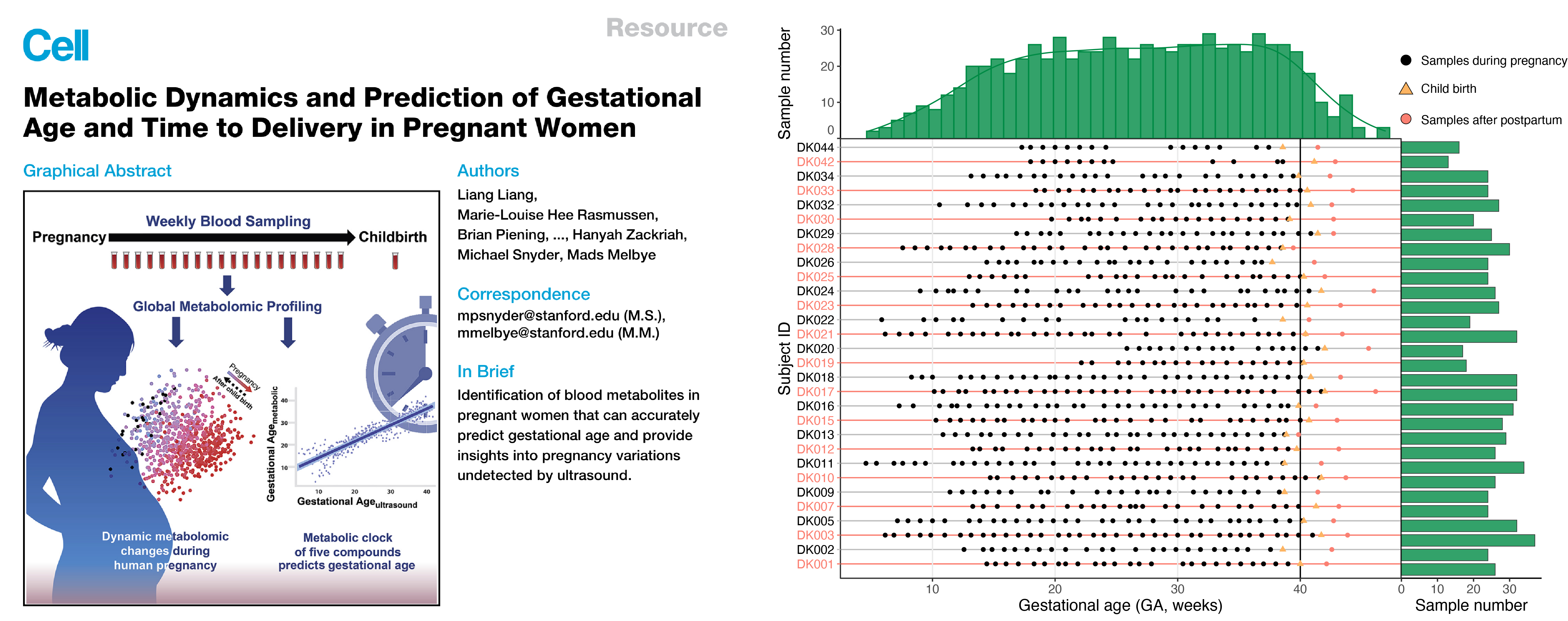### mzXML data

The LC-MS data (mzXML format) were deposited to the NIH Common Fund’s National Metabolomics Data Repository (NMDR) website, the Metabolomics Workbench, the project ID is PR000918 (https://doi.org/10.21228/M81H58).

### Pseudo-MS image datasets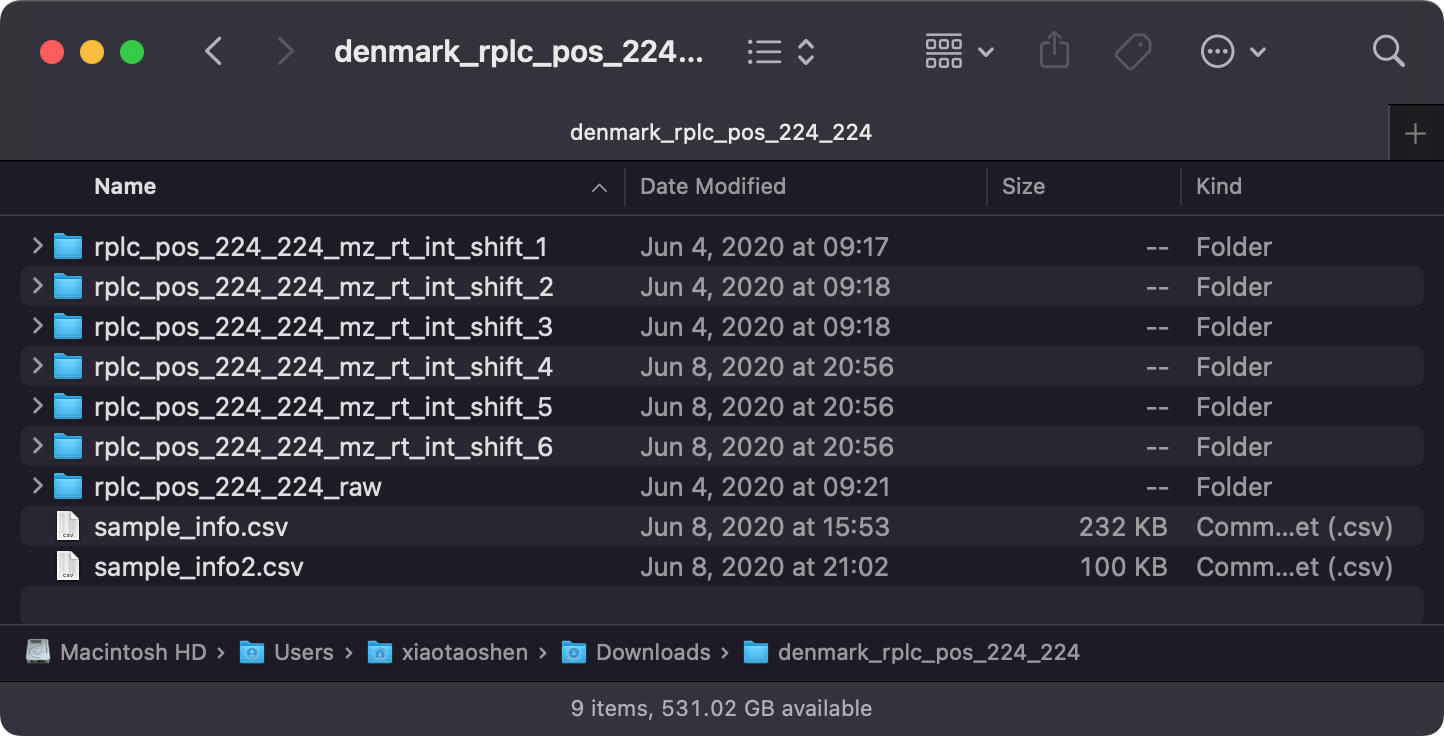`rplc_pos_224-224_raw` is the normal dataset (pseudo-MS images), and `rplc_pos_224-224_mz_rt_int_shift_x` are the augmented datasets by shift m/z, RT and intensity.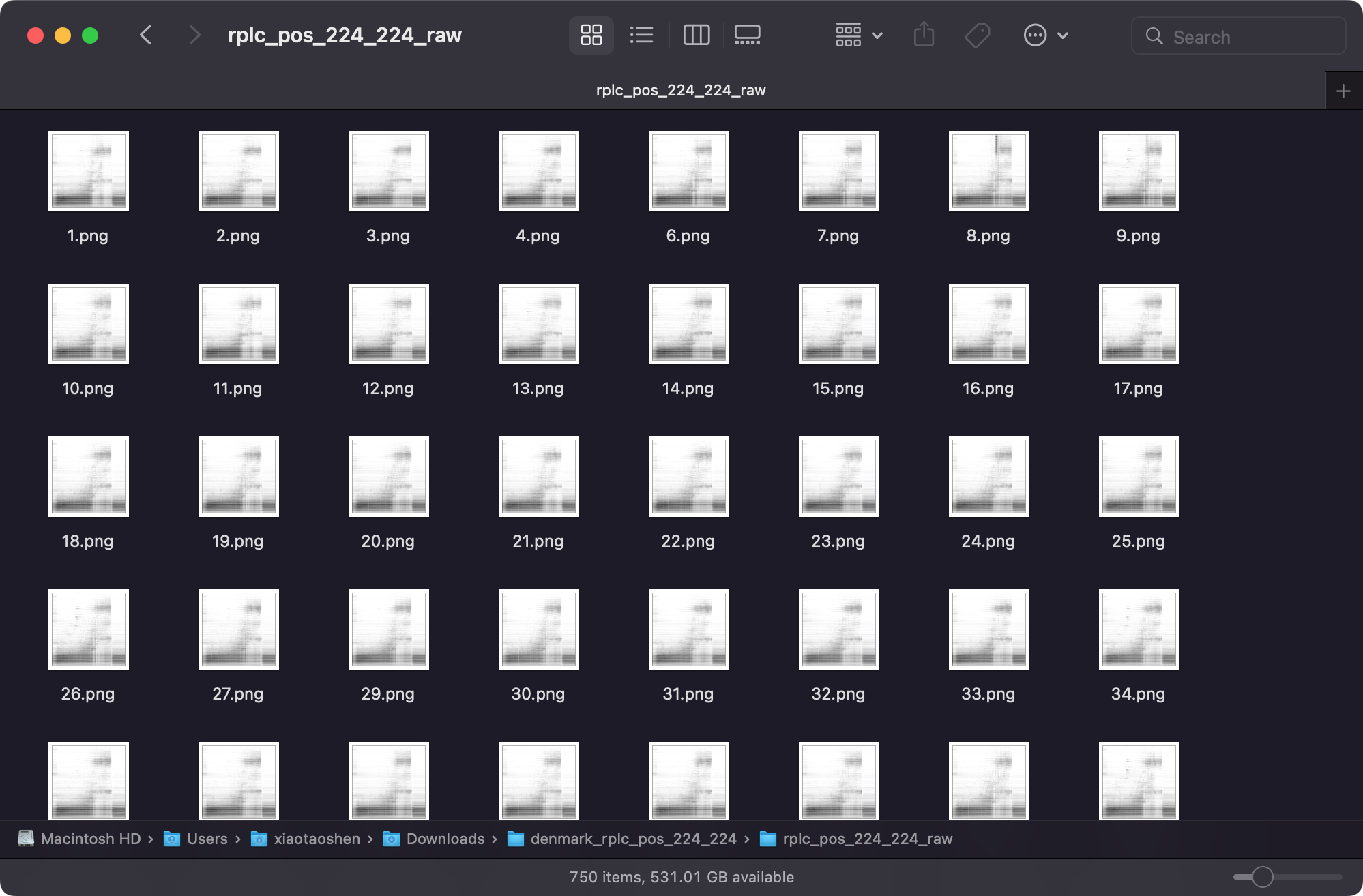## DeepPseduoMSI prediction result